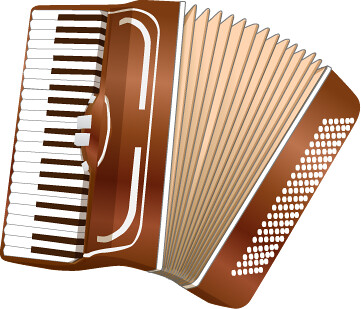hustf's blog

# Quantities in solving differential equations

This tutorial will introduce you to using quantities while solving Ordinary Differential Equations - ODEs, and with no reduction in calculation time compared to the 'pure numbers only' approach. We will be using and Julia, .

Traditionally, units are dropped from such calculations. Even calculation software with excellent unit support drops quantities in favour of pure numbers while solving differential equations.

The tutorial is loosely based on Differential equations.jl. There are indeed sections on units already. Here, we take relatable, unitful examples to show the benefits of keeping quantities in models. We use an adaption of the Unitful package as well as 'glue code' to other packages like Plots.jl.

### Input and output boxes

PS C:\Users\f> cd .julia\environments\
PS C:\Users\f\.julia\environments> mkdir temp
PS C:\Users\f\.julia\environments> cd temp
PS C:\Users\f\.julia\environments\temp>julia --project=.               _
_       _ _(_)_     |  Documentation: https://docs.julialang.org
(_)     | (_) (_)    |
_ _   _| |_  __ _   |  Type "?" for help, "]?" for Pkg help.
| | | | | | |/ _ |  |
| | |_| | | | (_| |  |  Version 1.6.0 (2021-03-24)
_/ |\__'_|_|_|\__'_|  |  Official https://julialang.org/ release
|__/                   |

julia> # Type ']' to change the julia>  prompt to install packages

Cloning registry from "https://github.com/hustf/M8"

(@v1.6) pkg> add MechanicalUnits, MechGluecode, DifferentialEquations, Revise

5 dependencies successfully precompiled in 37 seconds (214 already precompiled)

julia> 

We will drop the julia> promt from now on, so you can copy text more easily from the black boxes. If you restart the REPL, activate the same environment folder. And of course, you could use an editor or a notebook if you like.


Text output is shown in boxes with top border. Compilation messages & etc. are not shown.


### AccordionsSome code is for loading modules, which you may already have loaded. Other code just creates the graphics for this page. So we stow that code, like an accordion, with compressed bellows. Click '' to expand:

using Plots

plot(x-> rem(2x, 2cm), ribbon=30cm, xlims = (0, 15)cm, size = (200, 400))

# This is a trap; no plot here!
last_plot_with_data_to_html()

### Quantities

So what do we mean by a quantity exactly?

A quantity represents an amount, one state, as opposed to a number of units of that thing. Saying 'with units' is easier than 'with quantity', but that simple term also may support the 'counting units' way of thinking, the one which makes engineers talk about 'kips' (an especially abhorrent misuse). I wish we had a nice everyday word for quantity, some word with one or two syllables, with no'q' in front and certainly no 's' behind. 'Metric' was a candidate that's falling out of use already, but here we are.

Finding clear definitions is not easy when just about everybody wants to use units. The plural form in many units indicates that we should be able to count an integer number of full bushels or stones or such. But a quantity, to us at least, is an idealized size, an unchanging platonic idea, a continuous measurement of something real which can only be described by picking some unit of the correct dimensionality, dividing the quantity by that unit, and presenting the resulting number and unit.

In our context, a vector or a tensor is not a quantity. We think of those as vectors and tensors consisting of quantities. A complex number multiplied by a unit is just one quantity.

Let's look at an example quantity, a certain length, and represent it in different units:

import MechanicalUnits: @import_expand, dimension
@import_expand(mm, cm, m, km, ft, inch, s, °, kg)

println( 1km .|> (m, ft, m²/ft ))
println([dimension(x) for x in (m, ft, m²/ft)])
(1000m, (1250000//381)ft, (1524//5)m²∙ft⁻¹)
[ ᴸ,  ᴸ,  ᴸ]


Yes, m²∙ft⁻¹ has dimension , short for length. So we were able to show the quantity which is best represented as 1 km in terms of that unit.

Maybe someone just realized:

Rounding a quantity to a number of digits has no meaning. You can only round a quantity in terms of a unit.

Hence, round(ft, 1km) =3281 ft

The type Quantity that we use has

1. A number. Usually, a Float64 has enough digits.

2. Dimensionality.

3. Current unit, how we represent this quantity.

But there's really only two fixed parameters here: Dimensionality ("what it is") and size. This means that you could convert any quantity to a unit including, say, meter. But with any unit conversion, you don't get to pick the conversion factor. That factor is multiplied with your original numeric value. That conversion factor may itself be a quantity. In deed, that makes sense, and here's an example:

Measurers of strain might get excited, even worried, when strain exceeds 0.001. It's a dimensionless quantity, really, the lenght of change per unstrained length. But an expert would want to have more specific language in the long run. What they write instead of this number is μm/m . Which still has no dimensionality, and just means 10⁻⁶. When they talk? Just 'mu' of course.

Here, we only load the specific units we need. We are going to load a lot of code in the following, and having too many unit types in memory may trigger unwanted compilation. We don't want to compile methods for plotting candela or integrating pound. But for sketch calculations, using MechanicalUnits` is more practical. That will load the everyday units.

Next page: ODE

• Rackauckas, Christopher and Nie, Qing Differentialequations.jl–a performant and feature-rich ecosystem for solving differential equations in julia, Journal of Open Research Software, 5, 2017.

• Bezanson, Jeff and Edelman, Alan and Karpinski, Stefan and Shah, Viral B, SIAM review 59, 2017